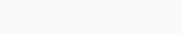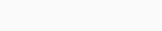# First Machine Learning Project in Python Step-By-Step

The best way to learn machine learning in Python by making small projects. Here, we take a small example of the machine learning project of linear regression. Before starting the project let understand machine learning and linear regression.

### Machine learning

In simple terms, Machine learning is the process in which machines (like a robot, computer) learns the things/algorithms to perform some tasks based on previous experience. Machine learning used in various places for example Tumor detection, Self-driving car, Recommendation system, etc.  There are mainly three types of machine learning.

1. Supervised learning
2. Unsupervised learning
3. Reinforcement learning.

### Linear regression

Linear regression is a technique of supervised learning. It is a statistical approach to find the relationship between variables. Linear regression mostly used for prediction.

## Making project in Machine learning

Here we make a project of linear regression. We make this project in four steps.

1. Implement libraries
3. Visualizing the data
4. Building the model

Step – 1 Implementing libraries

First, we need to import the necessary libraries of Python. Here, we use Pandas, Numpy, Sklearn libraries of python.

Numpy: Numpy is a Python package used for scientific calculation, for example performing different operations on matrix.

Sklearn: Sklearn is a Python package used for performing different machine learning operations, for example predicting the unknown future values.

Pandas: Pandas is a Python package used as a data analysis tool, easy use of data-structure, for example, Dataset can easily be analyzed by the plot.

```import pandas as pd
import numpy as np
import matplotlib.pyplot as plt
from sklearn.linear_model import LinearRegression
from sklearn.metrics import r2_score```

Step – 2 Reading the data

Here use one .csv file with random data. instead of this random data use the dataset.

```data=pd.read_csv('Sales.csv')
data```

Output:Step – 3 Visualizing the data

Describing the data by describe function.

`data.describe()`

Output:The graph between sales and month of the year.

`plt.scatter(data['Month_of_year'],data['Sales'])`

Output:Step – 4 Building the model

Splitting the data in input and target value.

```X=data[['Month_of_year']]
y=data['Sales']```

Making a linear regression model and fitting data into it.

```model=LinearRegression()
model.fit(X,y)```

Prediction by model

`y_pred=model.predict(X)`

A plot of linear regression.

```plt.scatter(X,y)
plt.plot(X,y_pred,color='Red')```

Output:Evaluating the model by computing the R square score.

`r2_score(y,y_pred)`

Output:Now, we predict the sales for 7.5 months by this model.

`print(model.predict([[7.5]]))`

Output:#### Dataset

Dataset used here is a random dataset created by me. You can also use another dataset. You can download a dataset from here: Sales.csv

#### Conclusion

With the help of this small project easily understand the following:

• Machine learning
• Linear regression

For many other projects or other important matters use of Machine learning and linear regression easy way analyze, predict, and get the result with accuracy.